# Test: Sinusoidal Steady‐State Analysis - 1

## 10 Questions MCQ Test Network Theory (Electric Circuits) | Test: Sinusoidal Steady‐State Analysis - 1

Description
Attempt Test: Sinusoidal Steady‐State Analysis - 1 | 10 questions in 30 minutes | Mock test for Electrical Engineering (EE) preparation | Free important questions MCQ to study Network Theory (Electric Circuits) for Electrical Engineering (EE) Exam | Download free PDF with solutions
QUESTION: 1

### i(t) = ?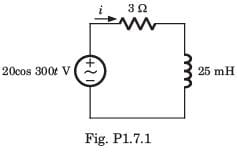Solution:

Z = 3 + j(25m)( 300) = 3 +  j7.5 Ω = 8.08     68.20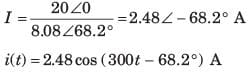QUESTION: 2

### vC(t) = ?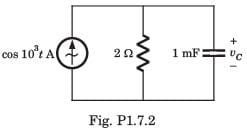Solution: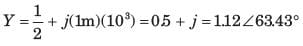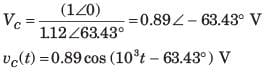QUESTION: 3

### vC(t) = ?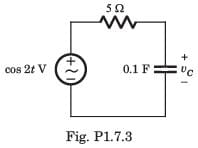Solution: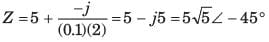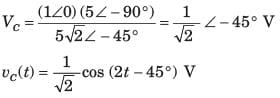QUESTION: 4

vC(t) = ?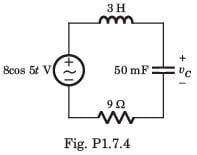Solution: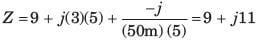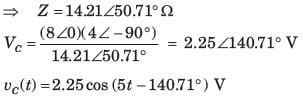QUESTION: 5

i(t) = ?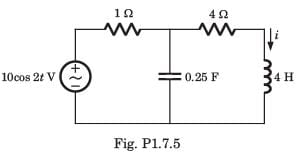Solution: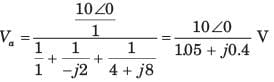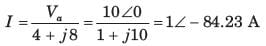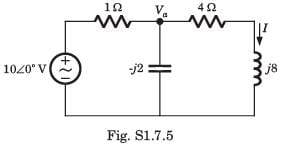i(t) = cos (2 t - 84.230) A

QUESTION: 6

In the bridge shown in fig. P1.7.13, Z1 = 300Ω, Z2= ( 300 - 600) Ω, Z3 = (200 + 100)Ω. The Z4 at balance is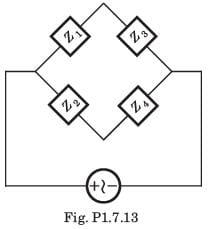Solution:

Z1Z4 = Z3Z2
300Z4 = (300-j600)(200+j100)
⇒ Z4 = 400 - j300

QUESTION: 7

In a two element series circuit, the applied voltage and the resulting current are v(t) = 60 + 66 sin (103t) V, i(t) = 2.3 sin (103t + 68.3.0) A. The nature of the elements would be

Solution:

R - C causes a positive phase shift in voltage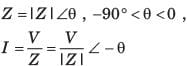QUESTION: 8

Vo = ?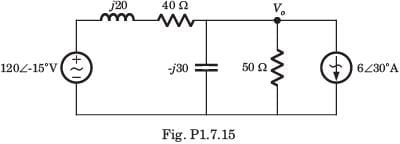Solution: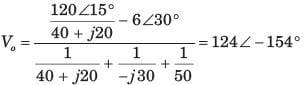QUESTION: 9

v0(t) = ?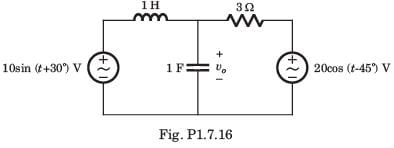Solution: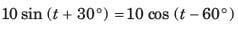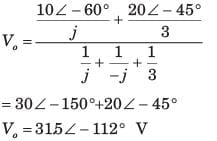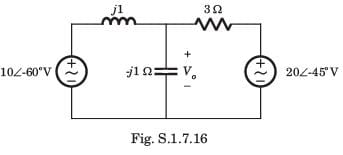QUESTION: 10

The circuit is as shown in fig. P1.7.17-18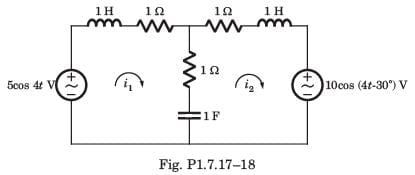Q. i1(t) = ?

Solution: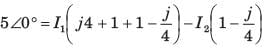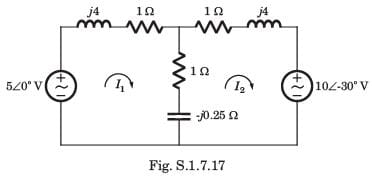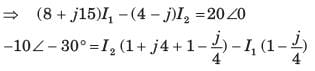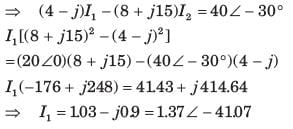Use Code STAYHOME200 and get INR 200 additional OFF Use Coupon Code